# inbreedR step by step

## inbreedR

The idea behind inbreedR is to provide a consistent framework for the analysis of inbreeding and heterozygosity-fitness correlations (HFCs) based on genetic markers. This vignette gives a practical introduction into the concept of the package and how to use the functions. For a more concise theoretical background and a citation, please refer to our paper (Stoffel et al. 2016). We are happy about any suggestions and feedback on the package. Just write a mail to martin.adam.stoffel[at]gmail.com.

## Installation

inbreedR is available on CRAN. Here is the code to download and install the current stable release.

install.packages("inbreedR")

The development version can be downloaded from GitHub with the following code:

install.packages("devtools")
devtools::install_github("mastoffel/inbreedR", build_vignettes = TRUE)

The package provides documentation for every function. To get an overview, just look at inbreedR’s help file.

library("inbreedR")
?inbreedR

## inbreedR contains the following functions:

• convert_raw: Converts a common format for genetic markers (two columns per locus) into the inbreedR working format, type ?convert_raw for detailed information.

• check_data: Checks whether the genotypes data.frame or matrix has the correct format for the inbreedR functions.

• sMLH: Computes standardized multilocus heterozygosities (Coltman et al. 1999).

• MLH: Computes multilocus heterozygosity.

• g2_microsats: Calculates $$g_2$$, a measure if identity disequlibrium (ID) from smaller datasets, such as microsatellites. Based on the formula from DAVID et al. (2007).

• g2_snps: Calculates $$g_2$$ for larger datasets, such as SNPs. Allows for parallelization to speed up computation times. Based on the formula from the appendix of J. I. Hoffman et al. (2014).

• HHC: Computes heterozygosity-heterozygosity correlations, another measure of identity disequilibrium (Balloux, Amos, and Coulson 2004).

• r2_Wf : Calculates the expected squared correlation of inbreeding-level (f) with a fitness trait (W) according to Szulkin, Bierne, and David (2010).

• r2_hf: Calculates the expected quared correlation of inbreeding-level (f) with multilocus heterozygosity (h) according to Szulkin, Bierne, and David (2010).

• simulate_g2: A simulation that allows the user to draw different numbers of markers independently from a simulated genome and calculate respective $$g_2$$ values. Can be used to evaluate the effects of the number of individuals and loci on the precision and magnitude of $$g_2$$.

• simulate_r2_hf: Works equivalent to simulate_g2. However the estimates are the expected squared correlations between inbreeding and heterozygosity $$r^2(h, f)$$.

• plot.inbreed: Plots for objects of class inbreed

## Example datasets

In the following sections, the functionality of inbreedR is illustrated using genetic and phenotypic data from an inbred captive population of oldfield mice (Peromyscus polionotus) (J. I. Hoffman et al. 2014). These mice were paired to produce offspring with a range of inbreeding coefficients (0-0.453) over six generations of laboratory breeding and the resulting pedigree was recorded, from which individual f values were calculated. Example files are provided containing the genotypes of 36 P. polionotus individuals at 12 microsatellites and 13,198 SNPs respectively. Data on body mass at weaning, a fitness proxy, are also available for the same individuals.

library(inbreedR)
data("mouse_msats") # microsatellite data
data("mouse_snps")  # snp data
data("bodyweight")  # fitness data

## Data format and checking

The working format of inbreedR is an individual * loci matrix or data frame in which rows represent individuals and each column represents a locus. If an individual is heterozygous at a given locus, it is coded as 1, whereas a homozygote is coded as 0, and missing data are coded as NA. The mouse_snps dataset accompanying the package is already formatted in the right way.

data("mouse_snps")
mouse_snps[1:10, 1:10]
#>    SNP1 SNP2 SNP3 SNP4 SNP5 SNP6 SNP7 SNP8 SNP9 SNP10
#> 11    0   NA   NA    0    0    0   NA    0    1     1
#> 22    0    0   NA    0    0    0    0    1    0     0
#> 32    0   NA   NA    0    0    0   NA   NA    1     0
#> 33    0    0    0    0    0    0    0    1    0     0
#> 34    1   NA   NA    1    0    0    0    0    0     0
#> 35    0   NA   NA    0    0    0   NA    0    1     0
#> 36    1    0   NA    0    0    0   NA    0    1     0
#> 1     1   NA   NA    0    0    0   NA    0    0     0
#> 2     0    0   NA    0    0    0   NA   NA    0     0
#> 3     0    0   NA    0    0    0   NA   NA    0     0

You can check whether your data is in the right format with the check_data function, which gives an error with a message when something went wrong and TRUE otherwise. Look up the documentation with ?check_data to see what exactly this functions checks for.

check_data(mouse_snps, num_ind = 36, num_loci = 13198)
#>  TRUE

### Conversion from a more common format

convert_raw is a function to convert a more common format, where each locus is represented by two columns (alleles), into the inbreedR working format. Microsatellite data is often formatted like mouse_msats, which is the second dataset accompanying the package.

data("mouse_msats")
mouse_msats[1:8, 1:8]
#>    Pml01.1 Pml01.2 Po3-68.1 Po3-68.2 Plgt58.1 Plgt58.2 Plgt62.1 Plgt62.2
#> 1       32      32       52       38       30       30       30       20
#> 2       14      14       20       20       36       24       30       30
#> 5       24      14       42       42       36       32       30       30
#> 6       14      14       40       20       32       32       38       30
#> 7       34      20       50       48       32       20       28       28
#> 8       14      14       42       38       32       10       38       28
#> 9       24      24       60       20       32       30       38       28
#> 10      32      20       46       38       30       30       30       20

To convert it into the inbreedR working format, just use the convert_raw function.

mouse_microsats <- convert_raw(mouse_msats)
mouse_microsats[1:8, 1:8]
#>    V1 V2 V3 V4 V5 V6 V7 V8
#> 1   0  1  0  1  1  1  1  0
#> 2   0  0  1  0  1  0  1  1
#> 5   1  0  1  0  0  0  0  0
#> 6   0  1  0  1  1  0  1  1
#> 7   1  1  1  0  0  1  1  1
#> 8   0  1  1  1  1  0  1  1
#> 9   0  1  1  1  1  1  1  0
#> 10  1  1  0  1  0  1  1  0

The same procedure works when you have letters (e.g. basepairs ‘A’, ‘T’) in two adjacent columns instead of microsatellite allele lengths.

SNP data will naturally occur as VCF file after variant calling. Here is a short workflow how to load a VCF file into R with the vcfR package, extract the genotypes and transform them into a 0/1 format to be used within inbreedR.

# install.packages("vcfR")
# install.packages("reshape")
library(vcfR)
library(reshape2)
vcf_file <- "yourvcffile.vcf"
vcf <- read.vcfR(vcf_file, verbose = FALSE )
# extract genotypes
gt <- extract.gt(vcf)
# transpose and data.frame
gt <- as.data.frame(t(gt), stringsAsFactors = FALSE)
# NA handling
gt[gt == "."] <- NA
# split columns
snp_geno <- do.call(cbind, apply(gt, 2, function(x) colsplit(x, "/", c("a","b"))))
# convert
mouse_snp_genotypes <- inbreedR::convert_raw(snp_geno)
# check data
check_data(mouse_snp_genotypes)

## A short theory of heterozygosity-fitness correlations (HFC)

Most HFC studies solely report the correlation between heterozygosity (h) and fitness (W). However, according to HFC theory, this correlation results from the simultaneous effects of inbreeding level (f) on fitness ($$r(W,f)$$) and heterozygosity ($$r(h,f$$)) (Slate et al. 2004; Szulkin, Bierne, and David 2010):

$r(W,h) = r(h,f)r(W,f)$ (Equation 1)

Although we cannot directly measure the inbreeding level f, we can use the extent to which heterozygosity is correlated across loci, termed identity disequilibrium (ID), as a proxy to characterize the distribution of f in populations. A measure of ID that can be related to HFC theory is the two-locus heterozygosity disequilibrium, $$g_2$$ (DAVID et al. 2007), which quantifies the extent to which heterozygosities are correlated across pairs of loci. Based on $$g_2$$ as an estimate of ID, it is then possible to calculate $$\hat{r}^2(h, f)$$ as follows (Szulkin, Bierne, and David 2010):

$\hat{r}^2(h, f) = \frac{\hat{g}_{2}}{\hat{\sigma}^2(h)}$ (Equation 2)

Finally, the expected determination coefficient between a fitness trait and inbreeding level can simply be derived be rearranging equation 1 (Szulkin, Bierne, and David 2010):

$\hat{r}^2(W, f) = \frac{\hat{r}^2(W, h)}{\hat{r}^2(h, f)}$ (Equation 3)

Software is already available for calculating $$g_2$$ from microsatellite datasets (DAVID et al. 2007). However, for larger datasets, e.g. SNPs, the original formula is not computationally practical, as it requires a double summation over all pairs of loci. For example, with 15.000 loci, the double summations take of the order of 0.2 x 109 computation steps. For this reason, inbreedR implements a computationally more feasible formula based on the assumption that missing values do not vary much between pairs of loci (J. I. Hoffman et al. 2014). In turn, the $$g_2$$ parameter builds the foundation for the implementation of the above framework to analyse HFCs, which is recommended to be routinely computed in future HFC studies (Szulkin, Bierne, and David 2010).

## Identity disequilibrium

The package provides two functions to calculate $$g_2$$, a proxy for Identity disequilibrium, for both small datasets (e.g. microsatellites) and large datasets (e.g.SNPs).

• The g2_microsats function implements the formula given in DAVID et al. (2007).

• For large datasets, e.g. SNPs, the g2_snps function implements a computationally more feasible formula. This function also provides an additional argument for parallelization which distributes bootstrapping and permutation across cores. The results of both functions can be plotted as histograms with CIs.

Have a look at the help files with ?g2_microsats and ?g2_snps for more information on the formulas.

For both microsatellites and SNPs, inbreedR calculates confidence intervals by bootstrapping over individuals. It also permutes the genetic data to generate a P-value for the null hypothesis of no variance in inbreeding in the sample (i.e. $$g_2$$ = 0).

g2_mouse_microsats <- g2_microsats(mouse_microsats, nperm = 100, nboot = 100, CI = 0.95)
g2_mouse_snps <- g2_snps(mouse_snps, nperm = 100, nboot = 10,
CI = 0.95, parallel = FALSE, ncores = NULL)

To display a summary of the results just print the output of an inbreedR function.

g2_mouse_microsats
#>
#>
#> Calculation of identity disequilibrium with g2 for microsatellite data
#> ----------------------------------------------------------------------
#>
#> Data: 36 observations at 12 markers
#> Function call = g2_microsats(genotypes = mouse_microsats, nperm = 100, nboot = 100,     CI = 0.95)
#>
#> g2 = 0.02179805, se = 0.01752047
#>
#> confidence interval
#>         2.5%        97.5%
#> -0.005068067  0.059027818
#>
#> p (g2 > 0) = 0.11 (based on 100 permutations)

plot shows the distribution of bootstrap results including the confidence interval.

par(mfrow=c(1,2))
plot(g2_mouse_microsats, main = "Microsatellites",
col = "cornflowerblue", cex.axis=0.85)
plot(g2_mouse_snps, main = "SNPs",
col = "darkgoldenrod1", cex.axis=0.85)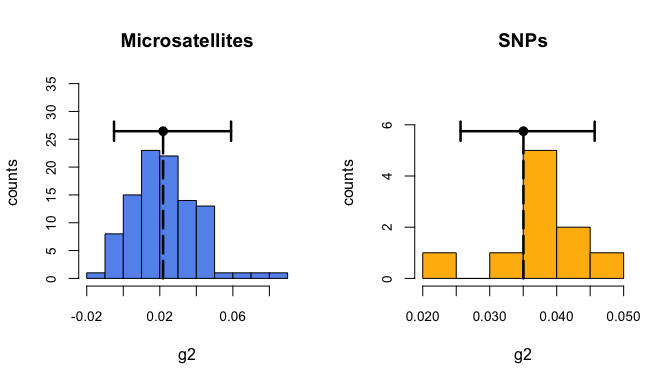Distribution of g2 from bootstrapping with confidence interval

Another approach for estimating ID is to divide the marker panel into two random subsets, compute the correlation in heterozygosity between the two, and repeat this hundreds or thousands of times in order to obtain a distribution of heterozygosity-heterozygosity correlation coefficients (HHCs) (Balloux, Amos, and Coulson 2004). This approach is intuitive but can be criticised on the grounds that samples within the HHC distribution are non-independent. Moreover, $$g_2$$ is preferable because it directly relates to HFC theory (equation 2). The HHC function in inbreedR calculates HHCs together with confidence intervals, specifying how often the dataset is randomly split into two halves with the reps argument. The results can be outputted as text or plotted as histograms with CIs.

HHC_mouse_microsats <- HHC(mouse_microsats , reps = 1000)
HHC_mouse_snps <- HHC(mouse_snps, reps = 100)
HHC_mouse_microsats
#>
#>
#> heterozygosity-heterozygosity correlations
#> ------------------------------------------
#>
#> Data: 36 observations at 12 markers
#> Function call = HHC(genotypes = mouse_microsats, reps = 1000)
#>
#> HHC Mean : 0.188
#> HHC SD: 0.125
#> HHC CI: [-0.041, 0.453]
par(mfrow=c(1,2))
plot(HHC_mouse_microsats, main = "Microsatellites",
col = "cornflowerblue", cex.axis=0.85)
plot(HHC_mouse_snps, main = "SNPs",
col = "darkgoldenrod1", cex.axis=0.85)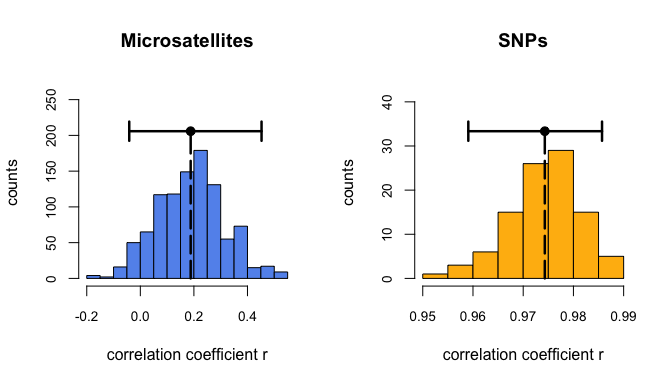Distribution of heterozygosity-heterozygosity correlations

## HFC parameters

Assuming that HFCs are due to inbreeding, it is possible to calculate both the expected correlation between heterozygosity and inbreeding level ($$\hat{r}^2(h, f)$$) and the expected correlation between a fitness trait and inbreeding ($$\hat{r}^2(W, f)$$) as described in Equation 1. These are implemented in inbreedR using the functions r2_hf and r2_Wf. Equal to the glm function, the distribution of the fitness trait can be specified in the family argument, as shown below:

# r^2 between inbreeding and heterozygosity
hf <- r2_hf(genotypes = mouse_microsats, type = "msats")
# r^2 between inbreeding and fitness
Wf <- r2_Wf(genotypes = mouse_microsats, trait = bodyweight,
family = gaussian, type = "msats")

In addition, bootstrapping over individuals can be used to estimate confidence intervals around these estimates. Also, there is the possibility of parallelization, by specifying parallel = TRUE

# r^2 between inbreeding and heterozygosity with bootstrapping
hf <- r2_hf(genotypes = mouse_microsats, nboot = 100, type = "msats", parallel = FALSE)

Plotting the histogram with confidence interval for r2_hf.

plot(hf)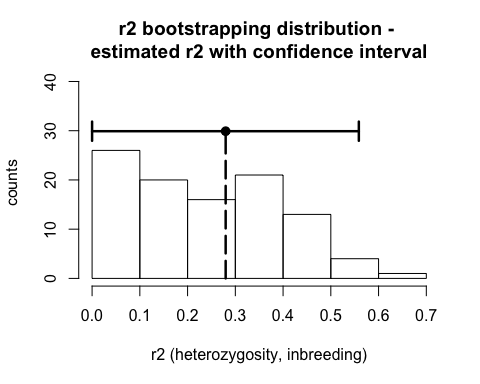## Workflow for estimating the impact of inbreeding on fitness using HFC

Szulkin, Bierne, and David (2010) in their online Appendix 1 provide a worked example of how to estimate the impact of inbreeding on fitness within an HFC framework. Below, we show how the required calculations can be implemented in inbreedR. We are now describing a coding workflow to estimate useful parameters for the interpretation of HFCs. We compare the results based on microsatellite and SNP data derived from a single inbred population of oldfield mice. We start with the estimation of identity disequilibrium ($$\hat{g}_2$$) and calculation of the distribution variance of standardized multilocus heterozygosity ($$\hat{\sigma}^2(h)$$), followed by the regression slope of fitness on heterozygosity ($$\hat{\beta}_{Wh}$$) and the three correlations from equation 1. Example code for the microsatellite dataset is shown below and the results for both microsatellites and SNPs are given in Table 1.

# g2
g2 <- g2_microsats(mouse_microsats)
# calculate sMLH
het <- sMLH(mouse_microsats)
# variance in sMLH
het_var <- var(het)
# Linear model of fitness trait on heterozygosity
mod <- lm(bodyweight ~ het)
# regression slope
beta <- coef(mod)
# r2 between fitness and heterozygosity
Wh <- cor(bodyweight,predict(mod))^2
# r2 between inbreeding and heterozygosity
hf <- r2_hf(genotypes = mouse_microsats, type = "msats")
# r2 between inbreeding and fitness
Wf <- r2_Wf(genotypes = mouse_microsats, trait = bodyweight,
family = gaussian, type = "msats")
Descriptors of HFCs
$$\hat{g}_2$$ $$\hat{\sigma}^2(h)$$ $$\hat{\beta}_{Wh}$$ $$\hat{r}^2_{Wh}$$ $$\hat{r}^2_{hf}$$ $$\hat{r}^2_{Wf}$$
microsats 0.022 0.078 1.601 0.121 0.28 0.434

## Sensitivity to the number of genetic markers

So far, the uncertainty of $$g_2$$ and other estimates is assessed via bootstrapping and confidence intervals. However, for planning future studies it might be of interest how the uncertainty of $$g_2$$ changes by increasing or decreasing the number of genetic markers.simulate_g2 can be used to evaluate the effects of the number of individuals and loci on the precision and magnitude of $$g_2$$. The user specifies the number of simulated individuals (n_ind), the subsets of loci (subsets) to be drawn, the heterozygosity of non-inbred individuals (H_nonInb) and the distribution of f among the simulated individuals. The f values of the simulated individuals are sampled randomly from a beta distribution with mean (meanF) and variance (varF) specified by the user (e.g. as in (Wang 2011)). This enables the simulation to mimic populations with known inbreeding characteristics, or to simulate hypothetical scenarios of interest. For computational simplicity, allele frequencies are assumed to be constant across all loci and the simulated loci are unlinked. Genotypes (i.e. the heterozygosity/homozygosity status at each locus) are assigned stochastically based on the f values of the simulated individuals. Specifically, the probability of an individual being heterozygous at any given locus ($$H$$) is expressed as $$H = H0(1-\f)$$ , where $$H0$$ is the user-specified heterozygosity of a non-inbred individual and f is an individual’s inbreeding coefficient drawn from the beta distribution. The type argument specifies the $$g_2$$ formula to use. With type = snps, simulations with larger loci sets are possible. However, bear in mind that the function creates independent loci for every repition, which leads to a rapid increase in working memory use and computation time.

sim_g2 <- simulate_g2(n_ind = 20, H_nonInb = 0.5, meanF = 0.2, varF = 0.05,
subsets = c(4,6,8,10,12), reps = 100,
type = "msats", CI = 0.95)

The results can easily be plotted with the plot function again.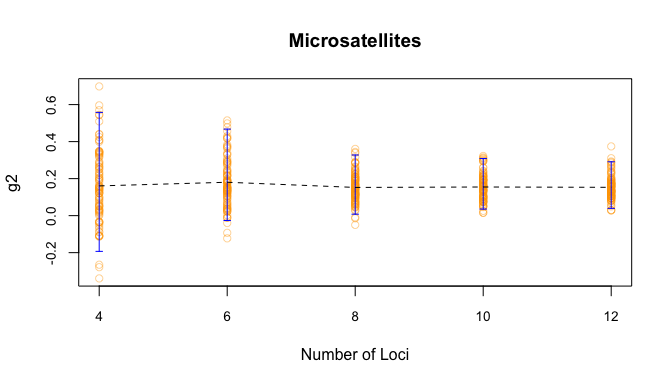Simulation: Sensitivity of g2 estimated from an increasing number of markers

Also, the plot function allows to plot the real $$g_2$$ value, which is directly computed from the realized inbreeding values of the individuals.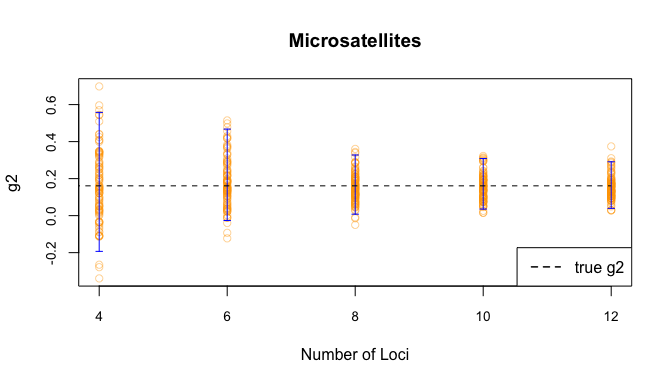Simulation: Sensitivity of g2 estimated from an increasing number of markers with true g2 value

## Note on calculating g2 for real-world SNP datasets

SNP datasets obtained by most sequencing approaches will yield a high proportion of missing data. It is important to be aware that the $$g_2$$ formula for SNPs is fast because it relies on the assumption that missing values don’t vary much between loci. We thus advice to calculate $$g_2$$ for datasets which are as complete as possible. In many situations, it might therefore be necessary to reduce the amount of SNPs by filtering for SNPs which have been genotyped in most individuals. Calculating confidence intervals around $$g_2$$ in combination with estimating the correlation between marker heterozygosity and inbreeding with the $$r2_hf$$ function will give you insights on whether the SNP density is sufficient to estimate $$g_2$$. Also, Huisman et al. (2016) show how to combine $$g_2$$ and other inbreeding estimates in the framework described above, thereby giving a good guideline for future studies.

## Extracting raw data from inbreedR objects

You may wish to extract and plot the data yourself. Most function outputs are inbreed objects and lists. In the Value section of each functions documentation (?fun ), you can see the data which you can extract. Alternatively, use str() to look at the object’s structure. Just index the function output with [["."]] or $ as in the following example: Running the function. g2_seals <- g2_microsats(mouse_microsats, nperm = 100, nboot = 100, CI = 0.95) Looking at the structure. str(g2_seals) #> List of 9 #>$ call     : language g2_microsats(genotypes = mouse_microsats, nperm = 100, nboot = 100,      CI = 0.95)
#>  $g2 : num 0.0218 #>$ p_val    : num 0.11
#>  $g2_permut: num [1:100] 0.021798 0.030426 0.043832 -0.000203 0.01776 ... #>$ g2_boot  : num [1:100] 0.0218 0.0241 0.0312 0.0261 0.0317 ...
#>  $CI_boot : Named num [1:2] -0.00648 0.05958 #> ..- attr(*, "names")= chr [1:2] "2.5%" "97.5%" #>$ g2_se    : num 0.017
#>  $nobs : int 36 #>$ nloc     : int 12
#>  - attr(*, "class")= chr "inbreed"

Now extract whatever you want from the object, such as the $$g_2$$ bootstrap results.

g2_bootstrap_results <- g2_seals\$g2_boot
str(g2_bootstrap_results)
#>  num [1:100] 0.0218 0.0241 0.0312 0.0261 0.0317 ...

## Literature

Balloux, F, W Amos, and T Coulson. 2004. “Does Heterozygosity Estimate Inbreeding in Real Populations?” Molecular Ecology 13 (10). Wiley Online Library: 3021–31.

Coltman, David W, Jill G Pilkington, Judith A Smith, and Josephine M Pemberton. 1999. “Parasite-Mediated Selection Against Inbred Soay Sheep in a Free-Living, Island Population.” Evolution, no. 53(4. JSTOR: 1259–67.

DAVID, PATRICE, BENOÎT PUJOL, FRÉDÉRIQUE VIARD, VINCENT CASTELLA, and JÉRÔME GOUDET. 2007. “Reliable selfing rate estimates from imperfect population genetic data.” Molecular Ecology 16 (12): 2474.

Hoffman, J I, F Simpson, P David, J M Rijks, T Kuiken, M A S Thorne, R C Lacy, and K K Dasmahapatra. 2014. “High-throughput sequencing reveals inbreeding depression in a natural population.” Proceedings of the National Academy of Sciences 111 (10): 3775–80.

Huisman, Jisca, Loeske EB Kruuk, Philip A Ellis, Tim Clutton-Brock, and Josephine M Pemberton. 2016. “Inbreeding Depression Across the Lifespan in a Wild Mammal Population.” Proceedings of the National Academy of Sciences 113 (13). National Acad Sciences: 3585–90.

Slate, J, P David, K G Dodds, B A Veenvliet, B C Glass, T E Broad, and J C McEwan. 2004. “Understanding the relationship between the inbreeding coefficient and multilocus heterozygosity: theoretical expectations and empirical data.” Heredity 93 (3): 255.

Stoffel, Martin A, Mareike Esser, Marty Kardos, Emily Humble, Hazel Nichols, Patrice David, and Joseph I Hoffman. 2016. “InbreedR: An R Package for the Analysis of Inbreeding Based on Genetic Markers.” Methods in Ecology and Evolution. Wiley Online Library.

Szulkin, Marta, Nicolas Bierne, and Patrice David. 2010. “HETEROZYGOSITY-FITNESS CORRELATIONS: A TIME fOR REAPPRAISAL.” Evolution 64 (5). Wiley Online Library: 1202–17.

Wang, J. 2011. “A New Likelihood Estimator and Its Comparison with Moment Estimators of Individual Genome-Wide Diversity.” Heredity 107 (5). Nature Publishing Group: 433–43.